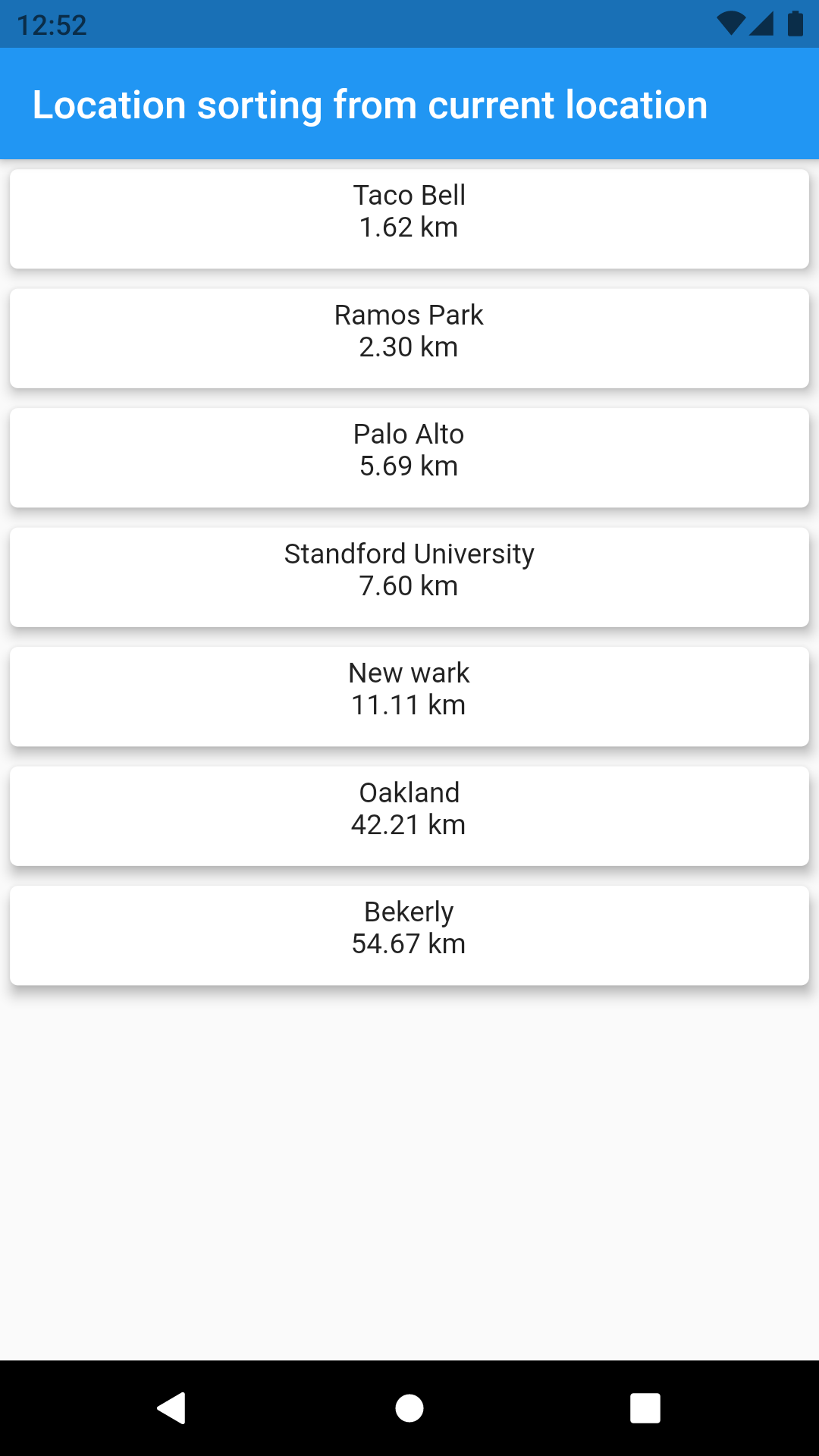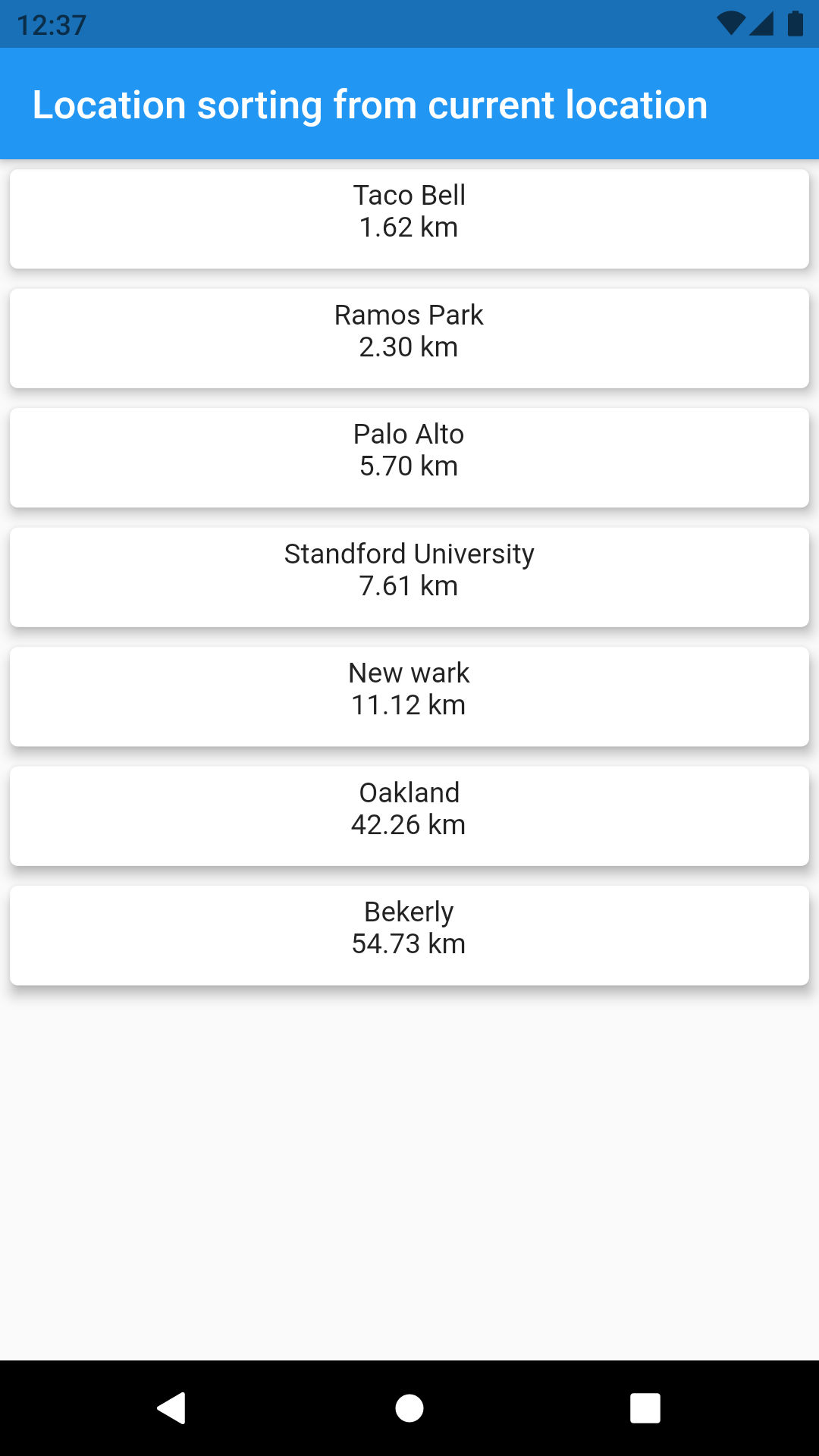Locations

Sorting of locations ref. nearest location.

Sort locations with nearest distance

How it works?

• Get user's current location
• Compare with other destinations
• Sort according the nearest

There are two ways to compare distance

Personally, I like Haversine Formula and have been using it.

Algorithm 1

Use Havesine Formula.

Haversine Formuladouble getDistanceFromLatLonInKm(lat1,lon1,lat2,lon2) {
var R = 6371; // Radius of the earth in km
var a =
Math.sin(dLat/2) * Math.sin(dLat/2) +
Math.sin(dLon/2) * Math.sin(dLon/2)
;
var c = 2 * Math.atan2(Math.sqrt(a), Math.sqrt(1-a));
var d = R * c; // Distance in km
return d;
}

return deg * (Math.pi/180);
}

Algorithm 2

Use inbuilt distance comparision function provided by Geolocater.

Geolocator.distanceBetween(source.latitude,source.longitude, destination.latitude,destination.longitude);

Assumption"

• You are in Google Plex (37.422,-122.084)

Destinations:

• Destination(37.4274684,-122.1698161, "Standford University"),
• Destination(37.4083327,-122.0776016, "Taco Bell"),
• Destination(37.4259071,-122.1095606, "Ramos Park"),
• Destination(37.8711583,-122.336457, "Bekerly"),
• Destination(37.7586968,-122.3053474, "Oakland"),
• Destination(37.4420794,-122.1432758, "Palo Alto"),
• Destination(37.5206515,-122.064364, "New wark")

Output:

Haversine Algorithm Geolocator AlgorithmGitHub

https://github.com/kapilmhr/sorting_nearest_location